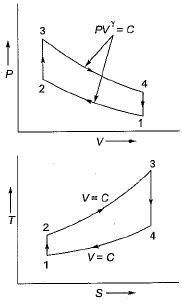Courses

# Test: Air Standard Cycle of IC Engine - 1

## 10 Questions MCQ Test Topicwise Question Bank for Mechanical Engineering | Test: Air Standard Cycle of IC Engine - 1

Description
This mock test of Test: Air Standard Cycle of IC Engine - 1 for Mechanical Engineering helps you for every Mechanical Engineering entrance exam. This contains 10 Multiple Choice Questions for Mechanical Engineering Test: Air Standard Cycle of IC Engine - 1 (mcq) to study with solutions a complete question bank. The solved questions answers in this Test: Air Standard Cycle of IC Engine - 1 quiz give you a good mix of easy questions and tough questions. Mechanical Engineering students definitely take this Test: Air Standard Cycle of IC Engine - 1 exercise for a better result in the exam. You can find other Test: Air Standard Cycle of IC Engine - 1 extra questions, long questions & short questions for Mechanical Engineering on EduRev as well by searching above.
QUESTION: 1

Solution:
QUESTION: 2

Solution:
QUESTION: 3

### Valve overlap is the period in which

Solution:
QUESTION: 4

Opening the intake valve before the TDC improves

Solution:
QUESTION: 5

Volumetric efficiency is not affected by

Solution:
QUESTION: 6

Mean effective pressure at a given compression ratio is maximum when the air-fuel ratio is

Solution: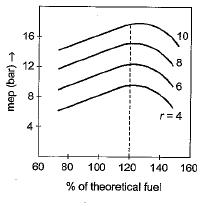When the air fuel ratio is lower than stoichiometric then only the mean effective pressure at a given compression ratio is maximum.

QUESTION: 7

The mean effective pressure of Otto cycle may be expressed in the form
(All notations have standard meaning)

Solution: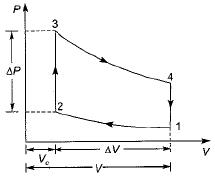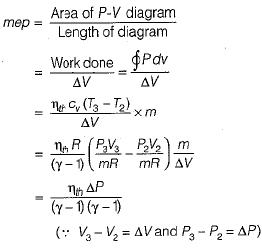QUESTION: 8

Lenoir cycle is used in

Solution:

Lenoir cycle consists of the following processes
• Constant volume heat addition (1-2)
• Constant pressure heat rejection (3-1)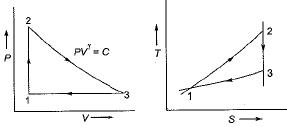Lenoir cycle is applicable to pulse jet engines.

QUESTION: 9

With increasing temperature of intake air, IC engine efficiency

Solution:

Due to high inlet temperature, maximum cycle temperature also increases which may cause dissociation.

QUESTION: 10

The air standard Otto cycle consists of

Solution:

Air standard Otto cycle consists of two reversible adiabatics, and two constant volume processor.